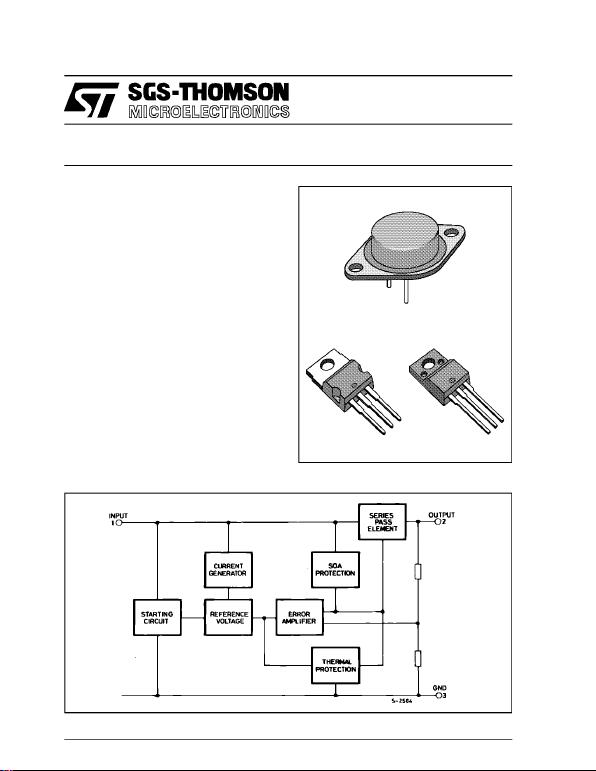L7800
SERIES
January 1993
POSITIVEVOLTAGE REGULATORS
.OUTPUT CURRENT UP TO 1.5A
.OUTPUTVOLTAGES OF 5; 5.2; 6; 8;8.5;9; 12;
15; 18;20; 24V
.THERMALOVERLOAD PROTECTION
.SHORT CIRCUIT PROTECTION
.OUTPUT TRANSISTOR SOA PROTECTION
DESCRIPTION
The L7800 series of three-terminal positive regula-
tor is available in TO-220, ISOWATT220 and TO-3
packages and with several fixed output voltages
making it useful in a wide range of applications.
These regulators can provide local on-card regula-
tion, eliminating the distribution problems associ-
atedwithsinglepointregulation. Eachtypeemploys
internal current limiting,thermalshut-down and safe
area protection, making it essentially indestructible.
Ifadequate heatsinkingisprovided, theycandeliver
over 1Aoutput current. Although designed primarily
as fixed voltage regulators, these devices can be
usedwith external components to obtainadjustable
voltages andcurrents.
BLOCK DIAGRAM
TO-3
TO-220 ISOWATT220
1/24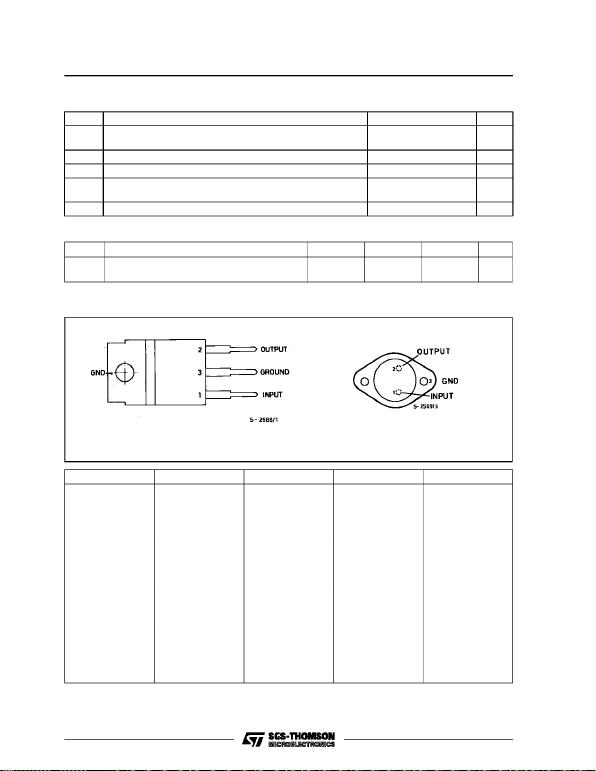ABSOLUTE MAXIMUM RATINGS
Symbol Parameter Value Unit
ViDC Input Voltage (for Vo= 5 to 18V)
(for Vo= 20, 24V) 35
40 V
V
IoOutput Current Internally limited
Ptot Power Dissipation Internally limited
Top Operating Junction Temperature (for L7800)
(for L7800C)–55to+150
0to+150 °C
°C
T
stg Storage Temperature 65 to + 150 °C
CONNECTION DIAGRAM AND ORDERING NUMBERS (top view)
THERMAL DATA
Symbol Parameter TO-220 ISOWATT220 TO-3 Unit
Rthj-case
Rthj-amb Thermal Resistance Junction-case Max
Thermal Resistance Junction-ambient Max 3
50 4
60 4
35
oC/W
oC/W
Type TO-220 ISOWATT220 TO-3 Output Voltage
L7805
L7805C
L7852C
L7806
L7806C
L7808
L7808C
L7885C
L7809C
L7812
L7812C
L7815
L7815C
L7818
L7818C
L7820
L7820C
L7824
L7824C
L7805CV
L7852CV
L7806CV
L7808CV
L7885CV
L7809CV
L7812CV
L7815CV
L7818CV
L7820CV
L7824CV
L7805CP
L7852CP
L7806CP
L7808CP
L7885CP
L7809CP
L7812CP
L7815CP
L7818CP
L7820CP
L7824CP
L7805T
L7805CT
L7852CT
L7806T
L7806CT
L7808T
L7808CT
L7885CT
L7809CT
L7812T
L7812CT
L7815T
L7815CT
L7818T
L7818CT
L7820T
L7820CT
L7824T
L7824CT
5V
5V
5.2V
6V
6V
8V
8V
8.5V
9V
12V
12V
15V
15V
18V
18V
20V
20V
24V
24V
TO-220 & ISOWATT220 TO-3
L7800 SERIES
2/24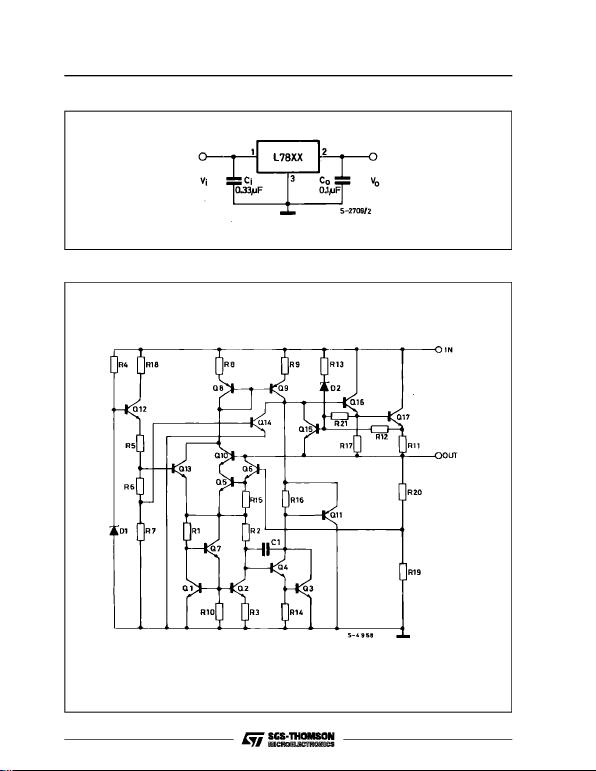APPLICATION CIRCUIT
SCHEMATIC DIAGRAM
L7800 SERIES
3/24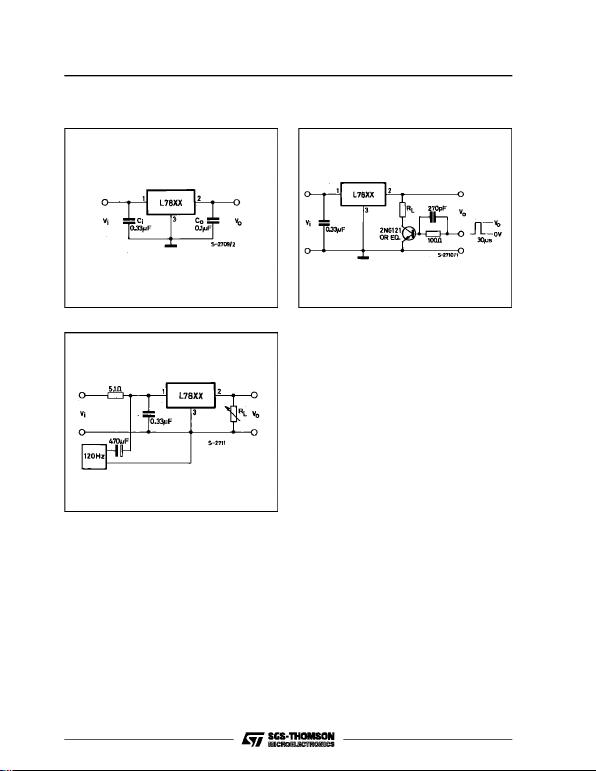TEST CIRCUITS
Figure 1 : DC Parameters. Figure 2 : Load Regulation.
Figure 3 : RippleRejection.
L7800 SERIES
4/24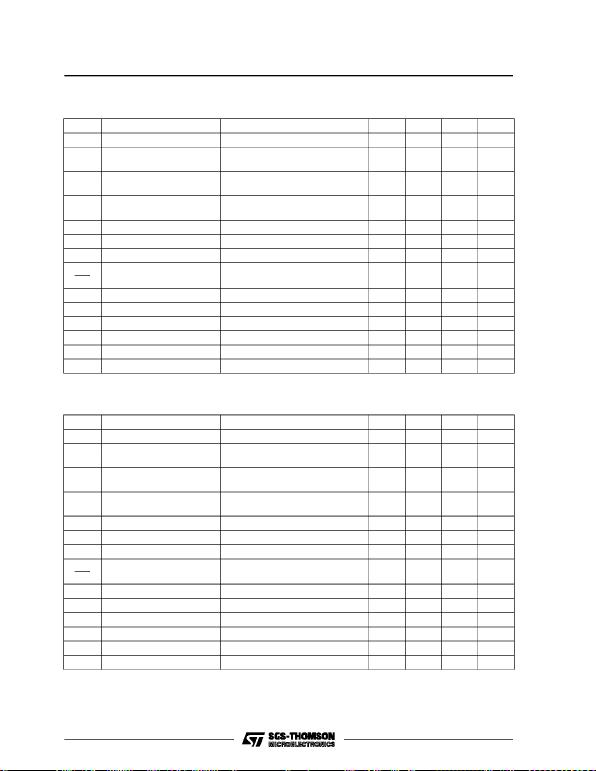* Load and line regulation are specified at constant junction temperature. Changes in Vodue to heating effects must be taken
into account separately. Pulce testing with low duty cycle is used.
ELECTRICAL CHARACTERISTICS FOR L7806 (refer to the test circuits, Tj=-55to150o
C,
Vi= 15V, Io= 500 mA, Ci=0.33µF, Co=0.1µF unless otherwise specified)
Symbol Parameter Test Conditions Min. Typ. Max. Unit
VoOutput Voltage Tj=25o
C 5.75 6 6.25 V
VoOutput Voltage Io= 5 mA to 1 A Po15 W
Vi= 9 to 21 V 5.65 6 6.35 V
Vo* Line Regulation Vi= 8 to 25 V Tj=25o
C
V
i= 9 to 13 V Tj=25o
C60
30 mV
mV
Vo* Load Regulation Io= 5 to 1500 mA Tj=25o
C
I
o= 250 to 750 mA Tj=25o
C100
30 mV
mV
IdQuiescent Current Tj=25o
C6mA
I
d
Quiescent Current Change Io= 5 to 1000 mA 0.5 mA
IdQuiescent Current Change Vi= 9 to 25 V 0.8 mA
V
o
T
Output Voltage Drift Io= 5 mA 0.7 mV/oC
eN Output Noise Voltage B = 10Hz to 100KHz Tj=25o
C40µV/VO
SVR Supply Voltage Rejection Vi= 9 to 19 V f = 120 Hz 65 dB
VdDropout Voltage Io=1A T
j=25o
C 2 2.5 V
RoOutput Resistance f = 1 KHz 19 m
Isc Short Circuit Current Vi=35V T
j=25o
C 0.75 1.2 A
Iscp Short Circuit Peak Current Tj=25o
C 1.3 2.2 3.3 A
ELECTRICAL CHARACTERISTICS FOR L7805 (refer to the test circuits, Tj=-55to150o
C,
Vi= 10V, Io= 500 mA, Ci=0.33µF, Co=0.1µF unless otherwise specified)
Symbol Parameter Test Conditions Min. Typ. Max. Unit
VoOutput Voltage Tj=25o
C 4.8 5 5.2 V
VoOutput Voltage Io= 5 mA to 1 A Po15 W
Vi= 8 to 20 V 4.65 5 5.35 V
Vo* Line Regulation Vi= 7 to 25 V Tj=25o
C
V
i= 8 to 12 V Tj=25o
C3
150
25 mV
mV
Vo* Load Regulation Io= 5 to 1500 mA Tj=25o
C
I
o= 250 to 750 mA Tj=25o
C100
25 mV
mV
IdQuiescent Current Tj=25o
C6mA
I
d
Quiescent Current Change Io= 5 to 1000 mA 0.5 mA
IdQuiescent Current Change Vi= 8 to 25 V 0.8 mA
V
o
T
Output Voltage Drift Io= 5 mA 0.6 mV/oC
eN Output Noise Voltage B = 10Hz to 100KHz Tj=25o
C40µV/VO
SVR Supply Voltage Rejection Vi= 8 to 18 V f = 120 Hz 68 dB
VdDropout Voltage Io=1A T
j=25o
C 2 2.5 V
RoOutput Resistance f = 1 KHz 17 m
Isc Short Circuit Current Vi=35V T
j=25o
C 0.75 1.2 A
Iscp Short Circuit Peak Current Tj=25o
C 1.3 2.2 3.3 A
L7800 SERIES
5/24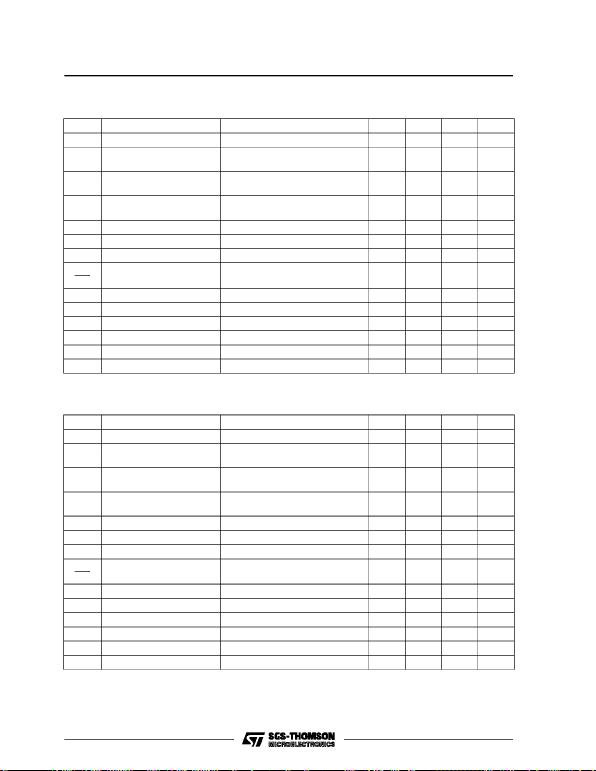* Load and line regulation are specified at constant junction temperature. Changes in Vodue to heating effects must be taken
into account separately. Pulce testing with low duty cycle is used.
ELECTRICAL CHARACTERISTICS FOR L7812 (refer to the test circuits, Tj=-55to150o
C,
Vi= 19V, Io= 500 mA, Ci=0.33µF, Co=0.1µF unless otherwise specified)
Symbol Parameter Test Conditions Min. Typ. Max. Unit
VoOutput Voltage Tj=25o
C 11.5 12 12.5 V
VoOutput Voltage Io= 5 mA to 1 A Po15 W
Vi= 15.5 to 27 V 11.4 12 12.6 V
Vo* Line Regulation Vi= 14.5 to 30 V Tj=25o
C
V
i=16to22V T
j=25o
C120
60 mV
mV
Vo* Load Regulation Io= 5 to 1500 mA Tj=25o
C
I
o= 250 to 750 mA Tj=25o
C100
60 mV
mV
IdQuiescent Current Tj=25o
C6mA
I
d
Quiescent Current Change Io= 5 to 1000 mA 0.5 mA
IdQuiescent Current Change Vi= 15 to 30 V 0.8 mA
V
o
T
Output Voltage Drift Io= 5 mA 1.5 mV/oC
eN Output Noise Voltage B = 10Hz to 100KHz Tj=25o
C40µV/VO
SVR Supply Voltage Rejection Vi= 15 to 25 V f = 120 Hz 61 dB
VdDropout Voltage Io=1A T
j=25o
C 2 2.5 V
RoOutput Resistance f = 1 KHz 18 m
Isc Short Circuit Current Vi=35V T
j=25o
C 0.75 1.2 A
Iscp Short Circuit Peak Current Tj=25o
C 1.3 2.2 3.3 A
ELECTRICAL CHARACTERISTICS FOR L7808 (refer to the test circuits, Tj=-55to150o
C,
Vi= 14V, Io= 500 mA, Ci=0.33µF, Co=0.1µF unless otherwise specified)
Symbol Parameter Test Conditions Min. Typ. Max. Unit
VoOutput Voltage Tj=25o
C 7.7 8 8.3 V
VoOutput Voltage Io= 5 mA to 1 A Po15 W
Vi= 11.5 to 23 V 7.6 8 8.4 V
Vo* Line Regulation Vi= 10.5 to 25 V Tj=25o
C
V
i=11to17V T
j=25o
C80
40 mV
mV
Vo* Load Regulation Io= 5 to 1500 mA Tj=25o
C
I
o= 250 to 750 mA Tj=25o
C100
40 mV
mV
IdQuiescent Current Tj=25o
C6mA
I
d
Quiescent Current Change Io= 5 to 1000 mA 0.5 mA
IdQuiescent Current Change Vi= 11.5 to 25 V 0.8 mA
V
o
T
Output Voltage Drift Io= 5 mA 1 mV/oC
eN Output Noise Voltage B = 10Hz to 100KHz Tj=25o
C40µV/VO
SVR Supply Voltage Rejection Vi= 11.5 to 21.5 V f = 120 Hz 62 dB
VdDropout Voltage Io=1A T
j=25o
C 2 2.5 V
RoOutput Resistance f = 1 KHz 16 m
Isc Short Circuit Current Vi=35V T
j=25o
C 0.75 1.2 A
Iscp Short Circuit Peak Current Tj=25o
C 1.3 2.2 3.3 A
L7800 SERIES
6/24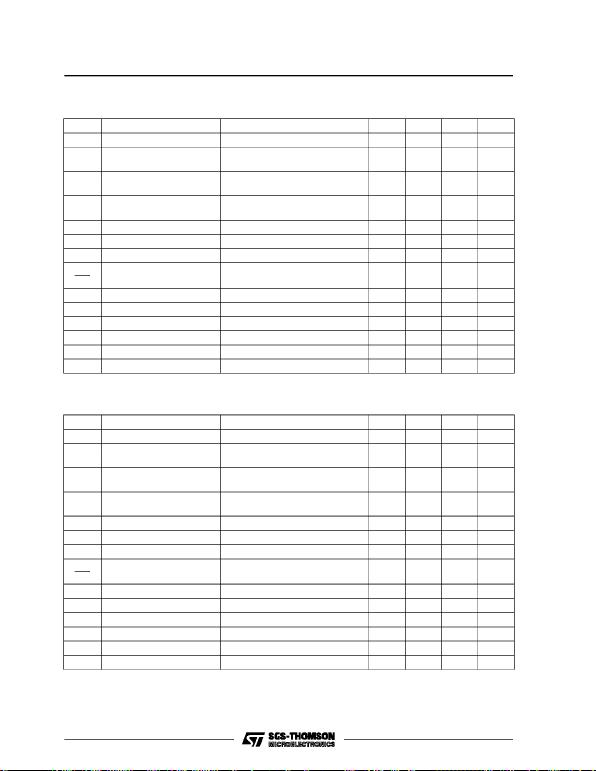* Load and line regulation are specified at constant junction temperature. Changes in Vodue to heating effects must be taken
into account separately. Pulce testing with low duty cycle is used.
ELECTRICAL CHARACTERISTICS FOR L7818 (refer to the test circuits, Tj=-55to150o
C,
Vi= 26V, Io= 500 mA, Ci=0.33µF, Co=0.1µF unless otherwise specified)
Symbol Parameter Test Conditions Min. Typ. Max. Unit
VoOutput Voltage Tj=25o
C 17.3 18 18.7 V
VoOutput Voltage Io= 5 mA to 1 A Po15 W
Vi=22to33V 17.1 18 18.9 V
Vo* Line Regulation Vi=21to33V T
j=25o
C
V
i=24to30V T
j=25o
C180
90 mV
mV
Vo* Load Regulation Io= 5 to 1500 mA Tj=25o
C
I
o= 250 to 750 mA Tj=25o
C180
90 mV
mV
IdQuiescent Current Tj=25o
C6mA
I
d
Quiescent Current Change Io= 5 to 1000 mA 0.5 mA
IdQuiescent Current Change Vi= 22 to 33 V 0.8 mA
V
o
T
Output Voltage Drift Io= 5 mA 2.3 mV/oC
eN Output Noise Voltage B = 10Hz to 100KHz Tj=25o
C40µV/VO
SVR Supply Voltage Rejection Vi= 22 to 32 V f = 120 Hz 59 dB
VdDropout Voltage Io=1A T
j=25o
C 2 2.5 V
RoOutput Resistance f = 1 KHz 22 m
Isc Short Circuit Current Vi=35V T
j=25o
C 0.75 1.2 A
Iscp Short Circuit Peak Current Tj=25o
C 1.3 2.2 3.3 A
ELECTRICAL CHARACTERISTICS FOR L7815 (refer to the test circuits, Tj=-55to150o
C,
Vi= 23V, Io= 500 mA, Ci=0.33µF, Co=0.1µF unless otherwise specified)
Symbol Parameter Test Conditions Min. Typ. Max. Unit
VoOutput Voltage Tj=25o
C 14.4 15 15.6 V
VoOutput Voltage Io= 5 mA to 1 A Po15 W
Vi= 18.5 to 30 V 14.25 15 15.75 V
Vo* Line Regulation Vi= 17.5 to 30 V Tj=25o
C
V
i=20to26V T
j=25o
C150
75 mV
mV
Vo* Load Regulation Io= 5 to 1500 mA Tj=25o
C
I
o= 250 to 750 mA Tj=25o
C150
75 mV
mV
IdQuiescent Current Tj=25o
C6mA
I
d
Quiescent Current Change Io= 5 to 1000 mA 0.5 mA
IdQuiescent Current Change Vi= 18.5 to 30 V 0.8 mA
V
o
T
Output Voltage Drift Io= 5 mA 1.8 mV/oC
eN Output Noise Voltage B = 10Hz to 100KHz Tj=25o
C40µV/VO
SVR Supply Voltage Rejection Vi= 18.5 to 28.5 V f = 120 Hz 60 dB
VdDropout Voltage Io=1A T
j=25o
C 2 2.5 V
RoOutput Resistance f = 1 KHz 19 m
Isc Short Circuit Current Vi=35V T
j=25o
C 0.75 1.2 A
Iscp Short Circuit Peak Current Tj=25o
C 1.3 2.2 3.3 A
L7800 SERIES
7/24* Load and line regulation are specified at constant junction temperature. Changes in Vodue to heating effects must be taken
into account separately. Pulce testing with low duty cycle is used.
ELECTRICAL CHARACTERISTICS FOR L7824 (refer to the test circuits, Tj=-55to150o
C,
Vi= 33V, Io= 500 mA, Ci=0.33µF, Co=0.1µF unless otherwise specified)
Symbol Parameter Test Conditions Min. Typ. Max. Unit
VoOutput Voltage Tj=25o
C 232425 V
V
o
Output Voltage Io= 5 mA to 1 A Po15 W
Vi=28to38V 22.8 24 25.2 V
Vo* Line Regulation Vi=27to38V T
j=25o
C
V
i=30to36V T
j=25o
C240
120 mV
mV
Vo* Load Regulation Io= 5 to 1500 mA Tj=25o
C
I
o= 250 to 750 mA Tj=25o
C240
120 mV
mV
IdQuiescent Current Tj=25o
C6mA
I
d
Quiescent Current Change Io= 5 to 1000 mA 0.5 mA
IdQuiescent Current Change Vi= 28 to 38 V 0.8 mA
V
o
T
Output Voltage Drift Io= 5 mA 3 mV/oC
eN Output Noise Voltage B = 10Hz to 100KHz Tj=25o
C40µV/VO
SVR Supply Voltage Rejection Vi= 28 to 38 V f = 120 Hz 56 dB
VdDropout Voltage Io=1A T
j=25o
C 2 2.5 V
RoOutput Resistance f = 1 KHz 28 m
Isc Short Circuit Current Vi=35V T
j=25o
C 0.75 1.2 A
Iscp Short Circuit Peak Current Tj=25o
C 1.3 2.2 3.3 A
ELECTRICAL CHARACTERISTICS FOR L7820 (refer to the test circuits, Tj=-55to150o
C,
Vi= 28V, Io= 500 mA, Ci=0.33µF, Co=0.1µF unless otherwise specified)
Symbol Parameter Test Conditions Min. Typ. Max. Unit
VoOutput Voltage Tj=25o
C 19.2 20 20.8 V
VoOutput Voltage Io= 5 mA to 1 A Po15 W
Vi=24to35V 19 20 21 V
Vo* Line Regulation Vi= 22.5 to 35 V Tj=25o
C
V
i=26to32V T
j=25o
C200
100 mV
mV
Vo* Load Regulation Io= 5 to 1500 mA Tj=25o
C
I
o= 250 to 750 mA Tj=25o
C200
100 mV
mV
IdQuiescent Current Tj=25o
C6mA
I
d
Quiescent Current Change Io= 5 to 1000 mA 0.5 mA
IdQuiescent Current Change Vi= 24 to 35 V 0.8 mA
V
o
T
Output Voltage Drift Io= 5 mA 2.5 mV/oC
eN Output Noise Voltage B = 10Hz to 100KHz Tj=25o
C40µV/VO
SVR Supply Voltage Rejection Vi= 24 to 35 V f = 120 Hz 58 dB
VdDropout Voltage Io=1A T
j=25o
C 2 2.5 V
RoOutput Resistance f = 1 KHz 24 m
Isc Short Circuit Current Vi=35V T
j=25o
C 0.75 1.2 A
Iscp Short Circuit Peak Current Tj=25o
C 1.3 2.2 3.3 A
L7800 SERIES
8/24* Load and line regulation are specified at constant junction temperature. Changes in Vodue to heating effects must be taken
into account separately. Pulce testing with low duty cycle is used.
ELECTRICAL CHARACTERISTICS FOR L7852C (refer to the test circuits, Tj=0to125o
C,
Vi= 10V, Io= 500 mA, Ci=0.33µF, Co=0.1µF unless otherwise specified)
Symbol Parameter Test Conditions Min. Typ. Max. Unit
VoOutput Voltage Tj=25o
C 5.0 5.2 5.4 V
VoOutput Voltage Io= 5 mA to 1 A Po15 W
Vi= 8 to 20 V 4.95 5.2 5.45 V
Vo* Line Regulation Vi= 7 to 25 V Tj=25o
C
V
i= 8 to 12 V Tj=25o
C3
1105
52 mV
mV
Vo* Load Regulation Io= 5 to 1500 mA Tj=25o
C
I
o= 250 to 750 mA Tj=25o
C105
52 mV
mV
IdQuiescent Current Tj=25o
C8mA
I
d
Quiescent Current Change Io= 5 to 1000 mA 0.5 mA
IdQuiescent Current Change Vi= 7 to 25 V 1.3 mA
V
o
T
Output Voltage Drift Io= 5 mA -1.0 mV/oC
eN Output Noise Voltage B = 10Hz to 100KHz Tj=25o
C42µV
SVR Supply Voltage Rejection Vi= 8 to 18 V f = 120 Hz 61 dB
VdDropout Voltage Io=1A T
j=25o
C2V
R
o
Output Resistance f = 1 KHz 17 m
Isc Short Circuit Current Vi=35V T
j=25o
C 750 mA
Iscp Short Circuit Peak Current Tj=25o
C 2.2 A
ELECTRICAL CHARACTERISTICS FOR L7805C (refer to the test circuits, Tj=0to125o
C,
Vi= 10V, Io= 500 mA, Ci=0.33µF, Co=0.1µF unless otherwise specified)
Symbol Parameter Test Conditions Min. Typ. Max. Unit
VoOutput Voltage Tj=25o
C 4.8 5 5.2 V
VoOutput Voltage Io= 5 mA to 1 A Po15 W
Vi= 7 to 20 V 4.75 5 5.25 V
Vo* Line Regulation Vi= 7 to 25 V Tj=25o
C
V
i= 8 to 12 V Tj=25o
C3
1100
50 mV
mV
Vo* Load Regulation Io= 5 to 1500 mA Tj=25o
C
I
o= 250 to 750 mA Tj=25o
C100
50 mV
mV
IdQuiescent Current Tj=25o
C8mA
I
d
Quiescent Current Change Io= 5 to 1000 mA 0.5 mA
IdQuiescent Current Change Vi= 7 to 25 V 0.8 mA
V
o
T
Output Voltage Drift Io= 5 mA -1.1 mV/oC
eN Output Noise Voltage B = 10Hz to 100KHz Tj=25o
C40µV
SVR Supply Voltage Rejection Vi= 8 to 18 V f = 120 Hz 62 dB
VdDropout Voltage Io=1A T
j=25o
C2V
R
o
Output Resistance f = 1 KHz 17 m
Isc Short Circuit Current Vi=35V T
j=25o
C 750 mA
Iscp Short Circuit Peak Current Tj=25o
C 2.2 A
L7800 SERIES
9/24* Load and line regulation are specified at constant junction temperature. Changes in Vodue to heating effects must be taken
into account separately. Pulce testing with low duty cycle is used.
ELECTRICAL CHARACTERISTICS FOR L7808C (refer to the test circuits, Tj=0to125o
C,
Vi= 14V, Io= 500 mA, Ci=0.33µF, Co=0.1µF unless otherwise specified)
Symbol Parameter Test Conditions Min. Typ. Max. Unit
VoOutput Voltage Tj=25o
C 7.7 8 8.3 V
VoOutput Voltage Io= 5 mA to 1 A Po15 W
Vi= 10.5 to 25 V 7.6 8 8.4 V
Vo* Line Regulation Vi= 10.5 to 25 V Tj=25o
C
V
i=11to17V T
j=25o
C160
80 mV
mV
Vo* Load Regulation Io= 5 to 1500 mA Tj=25o
C
I
o= 250 to 750 mA Tj=25o
C160
80 mV
mV
IdQuiescent Current Tj=25o
C8mA
I
d
Quiescent Current Change Io= 5 to 1000 mA 0.5 mA
IdQuiescent Current Change Vi= 10.5 to 25 V 1 mA
V
o
T
Output Voltage Drift Io= 5 mA -0.8 mV/oC
eN Output Noise Voltage B = 10Hz to 100KHz Tj=25o
C52µV
SVR Supply Voltage Rejection Vi= 11.5 to 21.5 V f = 120 Hz 56 dB
VdDropout Voltage Io=1A T
j=25o
C2V
R
o
Output Resistance f = 1 KHz 16 m
Isc Short Circuit Current Vi=35V T
j=25o
C 450 mA
Iscp Short Circuit Peak Current Tj=25o
C 2.2 A
ELECTRICAL CHARACTERISTICS FOR L7806C (refer to the test circuits, Tj=0to125o
C,
Vi= 11V, Io= 500 mA, Ci=0.33µF, Co=0.1µF unless otherwise specified)
Symbol Parameter Test Conditions Min. Typ. Max. Unit
VoOutput Voltage Tj=25o
C 5.75 6 6.25 V
VoOutput Voltage Io= 5 mA to 1 A Po15 W
Vi= 8 to 21 V 5.7 6 6.3 V
Vo* Line Regulation Vi= 8 to 25 V Tj=25o
C
V
i= 9 to 13 V Tj=25o
C120
60 mV
mV
Vo* Load Regulation Io= 5 to 1500 mA Tj=25o
C
I
o= 250 to 750 mA Tj=25o
C120
60 mV
mV
IdQuiescent Current Tj=25o
C8mA
I
d
Quiescent Current Change Io= 5 to 1000 mA 0.5 mA
IdQuiescent Current Change Vi= 8 to 25 V 1.3 mA
V
o
T
Output Voltage Drift Io= 5 mA -0.8 mV/oC
eN Output Noise Voltage B = 10Hz to 100KHz Tj=25o
C45µV
SVR Supply Voltage Rejection Vi= 9 to 19 V f = 120 Hz 59 dB
VdDropout Voltage Io=1A T
j=25o
C2V
R
o
Output Resistance f = 1 KHz 19 m
Isc Short Circuit Current Vi=35V T
j=25o
C 550 mA
Iscp Short Circuit Peak Current Tj=25o
C 2.2 A
L7800 SERIES
10/24ELECTRICAL CHARACTERISTICS FOR L7809C (refer to the test circuits, Tj=0to125o
C,
Vi= 15V, Io= 500 mA, Ci=0.33µF, Co=0.1µF unless otherwise specified)
Symbol Parameter Test Conditions Min. Typ. Max. Unit
VoOutput Voltage Tj=25o
C 8.65 9 9.35 V
VoOutput Voltage Io= 5 mA to 1 A Po15 W
Vi= 11.5 to 26 V 8.55 9 9.45 V
Vo* Line Regulation Vi= 11.5 to 26 V Tj=25o
C
V
i=12to18V T
j=25o
C180
90 mV
mV
Vo* Load Regulation Io= 5 to 1500 mA Tj=25o
C
I
o= 250 to 750 mA Tj=25o
C180
90 mV
mV
IdQuiescent Current Tj=25o
C8mA
I
d
Quiescent Current Change Io= 5 to 1000 mA 0.5 mA
IdQuiescent Current Change Vi= 11.5 to 26 V 1 mA
V
o
T
Output Voltage Drift Io= 5 mA -1.0 mV/oC
eN Output Noise Voltage B = 10Hz to 100KHz Tj=25o
C70µV
SVR Supply Voltage Rejection Vi= 12 to 23 V f = 120 Hz 55 dB
VdDropout Voltage Io=1A T
j=25o
C2V
R
o
Output Resistance f = 1 KHz 17 m
Isc Short Circuit Current Vi=35V T
j=25o
C 400 mA
Iscp Short Circuit Peak Current Tj=25o
C 2.2 A
* Load and line regulation are specified at constant junction temperature. Changes in Vodue to heating effects must be taken
into account separately. Pulce testing with low duty cycle is used.
ELECTRICAL CHARACTERISTICS FOR L7885C (refer to the test circuits, Tj=0to125o
C,
Vi= 14.5V, Io=500mA,C
i=0.33µF, Co=0.1µF unless otherwise specified)
Symbol Parameter Test Conditions Min. Typ. Max. Unit
VoOutput Voltage Tj=25o
C 8.2 8.5 8.8 V
VoOutput Voltage Io= 5 mA to 1 A Po15 W
Vi=11to26V 8.1 8.5 8.9 V
Vo* Line Regulation Vi=11to27V T
j=25o
C
V
i= 11.5 to 17.5 V Tj=25o
C160
80 mV
mV
Vo* Load Regulation Io= 5 to 1500 mA Tj=25o
C
I
o= 250 to 750 mA Tj=25o
C160
80 mV
mV
IdQuiescent Current Tj=25o
C8mA
I
d
Quiescent Current Change Io= 5 to 1000 mA 0.5 mA
IdQuiescent Current Change Vi=11to27V 1 mA
V
o
T
Output Voltage Drift Io= 5 mA -0.8 mV/oC
eN Output Noise Voltage B = 10Hz to 100KHz Tj=25o
C55µV
SVR Supply Voltage Rejection Vi= 12 to 22 V f = 120 Hz 56 dB
VdDropout Voltage Io=1A T
j=25o
C2V
R
o
Output Resistance f = 1 KHz 16 m
Isc Short Circuit Current Vi=35V T
j=25o
C 450 mA
Iscp Short Circuit Peak Current Tj=25o
C 2.2 A
L7800 SERIES
11/24* Load and line regulation are specified at constant junction temperature. Changes in Vodue to heating effects must be taken
into account separately. Pulce testing with low duty cycle is used.
ELECTRICAL CHARACTERISTICS FOR L7815C (refer to the test circuits, Tj=0to125o
C,
Vi= 23V, Io= 500 mA, Ci=0.33µF, Co=0.1µF unless otherwise specified)
Symbol Parameter Test Conditions Min. Typ. Max. Unit
VoOutput Voltage Tj=25o
C 14.4 15 15.6 V
VoOutput Voltage Io= 5 mA to 1 A Po15 W
Vi= 17.5 to 30 V 14.25 15 15.75 V
Vo* Line Regulation Vi= 17.5 to 30 V Tj=25o
C
V
i=20to26V T
j=25o
C300
150 mV
mV
Vo* Load Regulation Io= 5 to 1500 mA Tj=25o
C
I
o= 250 to 750 mA Tj=25o
C300
150 mV
mV
IdQuiescent Current Tj=25o
C8mA
I
d
Quiescent Current Change Io= 5 to 1000 mA 0.5 mA
IdQuiescent Current Change Vi= 17.5 to 30 V 1 mA
V
o
T
Output Voltage Drift Io= 5 mA -1 mV/oC
eN Output Noise Voltage B = 10Hz to 100KHz Tj=25o
C90µV
SVR Supply Voltage Rejection Vi= 18.5 to 28.5 V f = 120 Hz 54 dB
VdDropout Voltage Io=1A T
j=25o
C2V
R
o
Output Resistance f = 1 KHz 19 m
Isc Short Circuit Current Vi=35V T
j=25o
C 230 mA
Iscp Short Circuit Peak Current Tj=25o
C 2.1 A
ELECTRICAL CHARACTERISTICS FOR L7812C (refer to the test circuits, Tj=0to125o
C,
Vi= 19V, Io= 500 mA, Ci=0.33µF, Co=0.1µF unless otherwise specified)
Symbol Parameter Test Conditions Min. Typ. Max. Unit
VoOutput Voltage Tj=25o
C 11.5 12 12.5 V
VoOutput Voltage Io= 5 mA to 1 A Po15 W
Vi= 14.5 to 27 V 11.4 12 12.6 V
Vo* Line Regulation Vi= 14.5 to 30 V Tj=25o
C
V
i=16to22V T
j=25o
C240
120 mV
mV
Vo* Load Regulation Io= 5 to 1500 mA Tj=25o
C
I
o= 250 to 750 mA Tj=25o
C240
120 mV
mV
IdQuiescent Current Tj=25o
C8mA
I
d
Quiescent Current Change Io= 5 to 1000 mA 0.5 mA
IdQuiescent Current Change Vi= 14.5 to 30 V 1 mA
V
o
T
Output Voltage Drift Io= 5 mA -1 mV/oC
eN Output Noise Voltage B = 10Hz to 100KHz Tj=25o
C75µV
SVR Supply Voltage Rejection Vi= 15 to 25 V f = 120 Hz 55 dB
VdDropout Voltage Io=1A T
j=25o
C2V
R
o
Output Resistance f = 1 KHz 18 m
Isc Short Circuit Current Vi=35V T
j=25o
C 350 mA
Iscp Short Circuit Peak Current Tj=25o
C 2.2 A
L7800 SERIES
12/24* Load and line regulation are specified at constant junction temperature. Changes in Vodue to heating effects must be taken
into account separately. Pulce testing with low duty cycle is used.
ELECTRICAL CHARACTERISTICS FOR L7820C (refer to the test circuits, Tj=0to125o
C,
Vi= 28V, Io= 500 mA, Ci=0.33µF, Co=0.1µF unless otherwise specified)
Symbol Parameter Test Conditions Min. Typ. Max. Unit
VoOutput Voltage Tj=25o
C 19.2 20 20.8 V
VoOutput Voltage Io= 5 mA to 1 A Po15 W
Vi=23to35V 19 20 21 V
Vo* Line Regulation Vi= 22.5 to 35 V Tj=25o
C
V
i=26to32V T
j=25o
C400
200 mV
mV
Vo* Load Regulation Io= 5 to 1500 mA Tj=25o
C
I
o= 250 to 750 mA Tj=25o
C400
200 mV
mV
IdQuiescent Current Tj=25o
C8mA
I
d
Quiescent Current Change Io= 5 to 1000 mA 0.5 mA
IdQuiescent Current Change Vi=23to35V 1 mA
V
o
T
Output Voltage Drift Io= 5 mA -1 mV/oC
eN Output Noise Voltage B = 10Hz to 100KHz Tj=25o
C 150 µV
SVR Supply Voltage Rejection Vi= 24 to 35 V f = 120 Hz 52 dB
VdDropout Voltage Io=1A T
j=25o
C2V
R
o
Output Resistance f = 1 KHz 24 m
Isc Short Circuit Current Vi=35V T
j=25o
C 180 mA
Iscp Short Circuit Peak Current Tj=25o
C 2.1 A
ELECTRICAL CHARACTERISTICS FOR L7818C (refer to the test circuits, Tj=0to125o
C,
Vi= 26V, Io= 500 mA, Ci=0.33µF, Co=0.1µF unless otherwise specified)
Symbol Parameter Test Conditions Min. Typ. Max. Unit
VoOutput Voltage Tj=25o
C 17.3 18 18.7 V
VoOutput Voltage Io= 5 mA to 1 A Po15 W
Vi=21to33V 17.1 18 18.9 V
Vo* Line Regulation Vi=21to33V T
j=25o
C
V
i=24to30V T
j=25o
C360
180 mV
mV
Vo* Load Regulation Io= 5 to 1500 mA Tj=25o
C
I
o= 250 to 750 mA Tj=25o
C360
180 mV
mV
IdQuiescent Current Tj=25o
C8mA
I
d
Quiescent Current Change Io= 5 to 1000 mA 0.5 mA
IdQuiescent Current Change Vi=21to33V 1 mA
V
o
T
Output Voltage Drift Io= 5 mA -1 mV/oC
eN Output Noise Voltage B = 10Hz to 100KHz Tj=25o
C 110 µV
SVR Supply Voltage Rejection Vi= 22 to 32 V f = 120 Hz 53 dB
VdDropout Voltage Io=1A T
j=25o
C2V
R
o
Output Resistance f = 1 KHz 22 m
Isc Short Circuit Current Vi=35V T
j=25o
C 200 mA
Iscp Short Circuit Peak Current Tj=25o
C 2.1 A
L7800 SERIES
13/24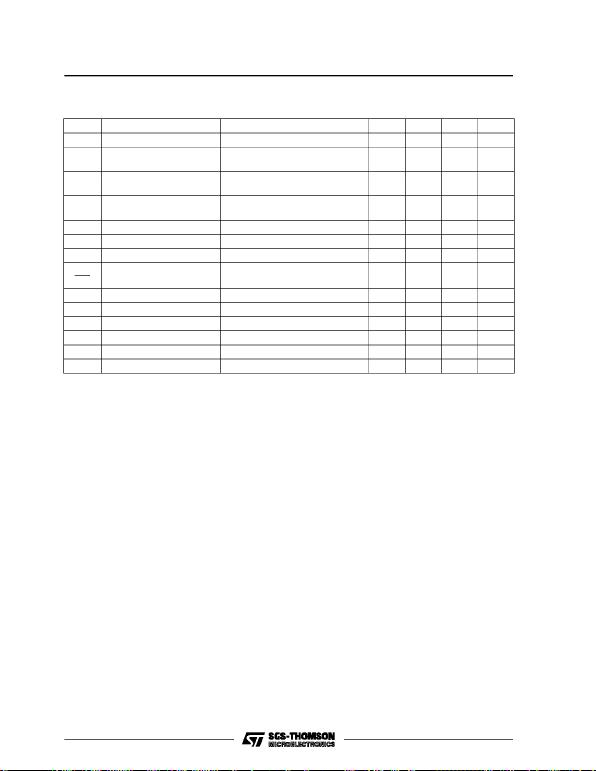* Load and line regulation are specified at constant junction temperature. Changes in Vodue to heating effects must be taken
into account separately. Pulce testing with low duty cycle is used.
ELECTRICAL CHARACTERISTICS FOR L7824C (refe</